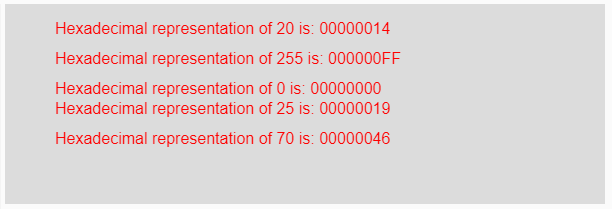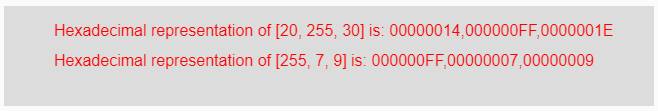# p5.js | hex() function

• Last Updated : 15 Apr, 2019

The hex() function in p5.js is used to convert a number into its hexadecimal notation.

Syntax:

Hey geek! The constant emerging technologies in the world of web development always keeps the excitement for this subject through the roof. But before you tackle the big projects, we suggest you start by learning the basics. Kickstart your web development journey by learning JS concepts with our JavaScript Course. Now at it's lowest price ever!

`hex(Number)`

Parameters: This function accepts a parameter Number which are to be converted into its hexadecimal form. This parameter might also be an array of numbers.

Return Value: It returns the converted hexadecimal representation.

Below program illustrates the hex() function in p5.js.
Example-1:

 `function` `setup() {`` ` `    ``// Creating Canvas size``    ``createCanvas(600, 200);``}`` ` `function` `draw() {`` ` `    ``// Set the background color ``    ``background(220);`` ` `    ``// Initializing some numbers``    ``let Number1 = 20;``    ``let Number2 = 255;``    ``let Number3 = 0;``    ``let Number4 = 25;``    ``let Number5 = 70;`` ` `    ``// Calling to hex() function.``    ``let A = hex(Number1);``    ``let B = hex(Number2);``    ``let C = hex(Number3);``    ``let D = hex(Number4);``    ``let E = hex(Number5);`` ` `    ``// Set the size of text ``    ``textSize(16);`` ` `    ``// Set the text color ``    ``fill(color(``'red'``));`` ` `    ``// Getting hexadecimal notation``    ``text(``"Hexadecimal representation of 20 is: "` `+ A, 50, 30);``    ``text(``"Hexadecimal representation of 255 is: "` `+ B, 50, 60);``    ``text(``"Hexadecimal representation of 0 is: "` `+ C, 50, 90);``    ``text(``"Hexadecimal representation of 25 is: "` `+ D, 50, 110);``    ``text(``"Hexadecimal representation of 70 is: "` `+ E, 50, 140);``}`

Output:Example-2:

 `function` `setup() {`` ` `    ``// Creating Canvas size``    ``createCanvas(650, 100);``}`` ` `function` `draw() {`` ` `    ``// Set the background color ``    ``background(220);`` ` `    ``// Initializing some numbers``    ``let Number1 = [20, 255, 30];``    ``let Number2 = [255, 7, 9];`` ` `    ``// Calling to hex() function.``    ``let A = hex(Number1);``    ``let B = hex(Number2);`` ` `    ``// Set the size of text ``    ``textSize(16);`` ` `    ``// Set the text color ``    ``fill(color(``'red'``));`` ` `    ``// Getting hexadecimal notation``    ``text(``"Hexadecimal representation of [20, 255, 30] is: "``     ``+ A, 50, 30);``    ``text(``"Hexadecimal representation of [255, 7, 9] is: "` `     ``+ B, 50, 60);``}`

Output:Reference: https://p5js.org/reference/#/p5/hex

My Personal Notes arrow_drop_up